# 41. gvSIG Geoprocesses. Vector layers¶

## 41.1. Group by a field

This geoprocess works over one unique input layer. The user will select a field for the association and the process will group the geometries according to the field chosen.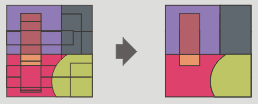The interface is: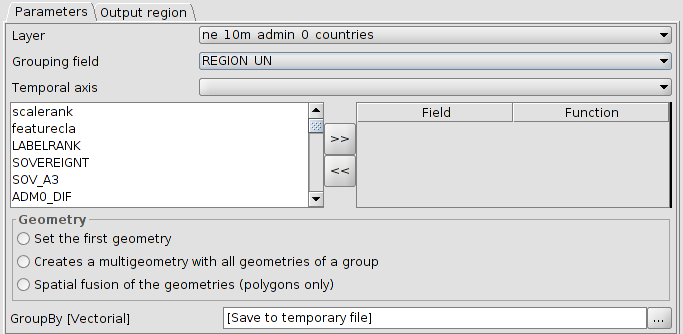1. Layer. To select the input layer from a dropdown menu.

2. Grouping field. To select the field from a dropdown menu.

3. Temporal axis. One particularity of this geoprocess is that it is possible to assign a time field if the input layer had it. In this case, the last or firs entry date can be included as a field table. This is very useful when the context table would be tables with sensor data, with many observations and all of them corresponding to an unique sensor. In this case is possible to group by the sensor type and assign to the table entry the last observation of the sensor. Also, we could select the option of assigning the first geometry having one point representing the sensor, with its data being the last made observation.

4. Field/Function. It is possible to include to the attribute table other fields. If they are numeric, a function can be applied and if they are text, assign them to the first ones.

5. Geometry. The output geometry can be:

• Set the first geometry. Only one of them (the first found).

• Creates a multigeometry with all geometries of a group.

• Spatial fusion of the geometries (polygons only).

## 41.2. Buffer

This geoprocess creates buffers around the geometries of vector features (points, lines and polygons) of one “input layer”, creating a new polygon vector layer.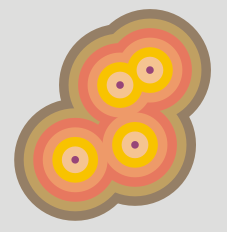The interface is: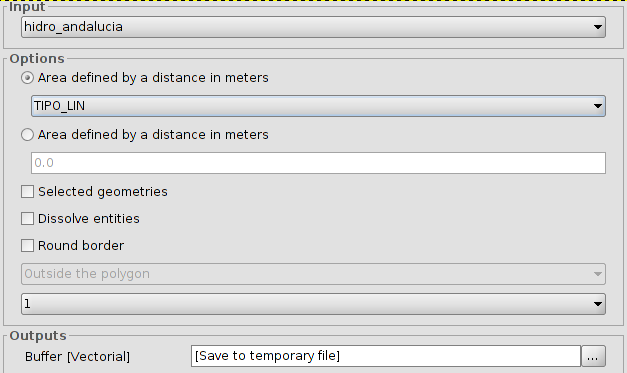1. Input. To select the input layer from a dropdown menu.

2. There are two options to define the area:

• Area defined by a field in meters: it is possible to select a field of the input layer, from which the buffer´s radius will be taken.

• Area defined by a distance in meters: it is possible to enter the numeric value of the buffer´s radius.

1. Selected geometries. Option to use only the selected features.

2. Dissolve entities (only one ring). It is possible to fuse the features of the buffer in contact.

3. Round border. Option to use a round border (soften).

4. In the case of polygonal features, the buffer can be outside the polygon, inside of the polygon, or both.

5. To set the number of buffers or equidistant rings around the input geometries.

If the input information is correct, when executing the geoprocess, a dialogue screen will show the progress, telling us the status in the buffer calculation. It is possible to cancel it at any time, clicking on "Cancel". In the case of the dissolve entities is not possible to calculate the exact duration, so the status bar will not be progressive. These process types are called “indefinites”.

When the geoprocess finishes, the new layer will be included in the TOC.

## 41.3. Lateral buffer

Creates a buffer in one of the sides of the feature (left or right).

The interface is: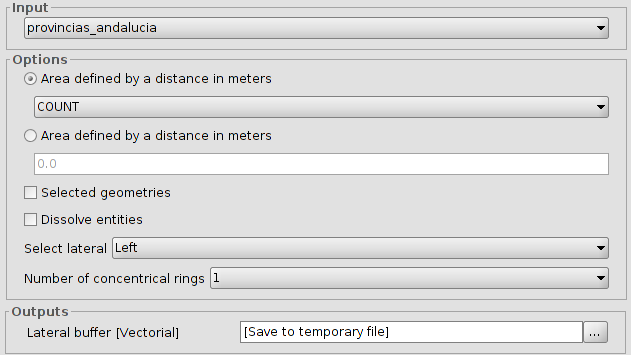1. Input. To select the input layer from a dropdown menu.

2. There are two options to define the area:

• Area defined by a field in meters: it is possible to select a field of the input layer, from which the buffer´s radius will be taken.

• Area defined by a distance in meters: it is possible to enter the numeric value of the buffer´s radius.

3. Selected geometries. Option to use only the selected features.

4. Dissolve entities (only one ring). It is possible to fuse the features of the buffer in contact.

5. Select lateral. To select the lateral to which the buffer will be made; left or right.

6. To set the number of buffers or equidistant rings around the input geometries.

If the input information is correct, when executing the geoprocess, a dialogue screen will show the progress, telling us the status in the lateral buffer calculation.

When the geoprocess finishes, the new layer will be included in the TOC.

## 41.4. Convex Hull

This geoprocess calculates the “Convex hull”, or convex polygon of the less area which involves all the vector elements of the “input layer”.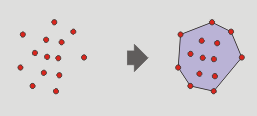The interface is: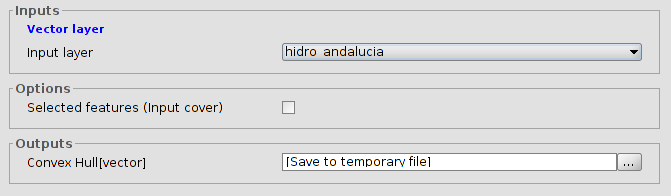1. Input layer.

2. Selected features (Input layer). Option to use only the selected features.

When the geoprocess finishes, the new layer will be included in the TOC.

## 41.5. Clip

To clip a vector layer (points, lines or polygons) using other layer as clip layer.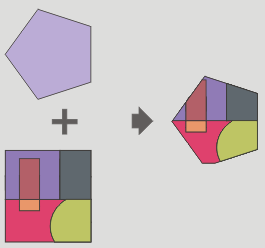The geoprocess will go over all the vector features of the input layer and with all of them inside of the area defined by the clip layer will calculate their intersections. In the output layer, only those will be present. The portion of feature out of the work area will be deleted.

The interface is: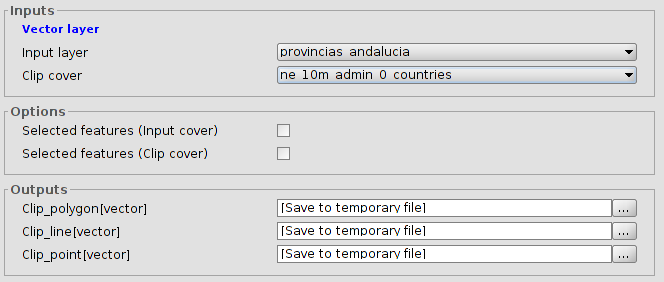1. Input layer. To select the layer to clip.

2. Clip cover. To select the layer to use as clip layer.

3. Selected features. Option to use only the selected features (input and clip layer)

as a result, a new layer with only the features inside of the area defined by the clip layer will be created. By default, three new output layers will be made, allowing to the user to discard any of them.

When the geoprocess finishes, the new layers will be included in the TOC.

## 41.6. Split lines

To split lines in equal size sections.

The interface is: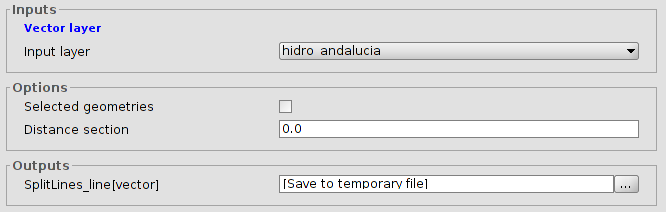1. Input layer.

2. Selected features. Option to use only the selected features.

3. Distance between sections. To set the size of the lines. The last part of the line has not to be the same that the defined distance.

When the geoprocess finishs, the new layer will be included in the TOC.

## 41.7. X and Y shift

To apply a shift change over all the points, lines and polygons of the input layer. For that, the shift in X and Y has to be defined.

The interface is: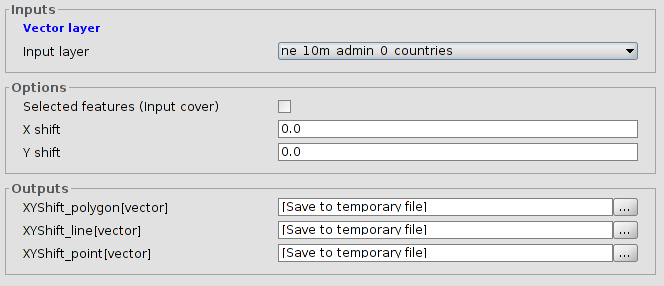1. Input layer.

2. Selected features (Input layer). Option to use only the selected features.

3. Shift in X and Y.

When the geoprocess finishes, the new layer will be included in the TOC.

## 41.8. Difference

This geoprocess known as “NOT Spatial” allows to get the areas of one layer that are not found in another layer. The features in both layers can be polygons, lines or points.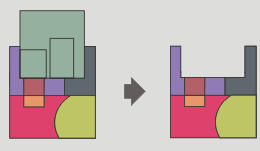The interface is: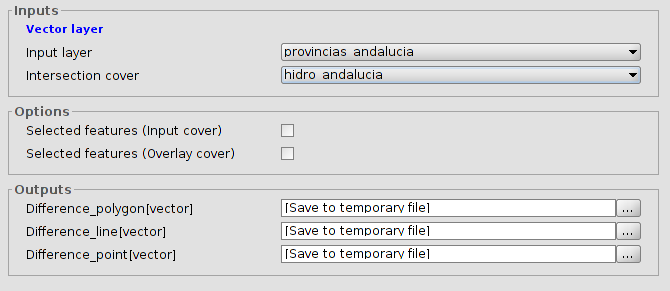1. Input layer.

2. Intersection cover.

3. Selected features. Option to use only the selected features in both layers.

The types of output layers will vary depending on the type of the input layers:

• Between a polygon layer and a linear one, the output layer will be polygonal.

• Between a linear layer and a polygonal one, the output layer will be linear.

• Between a polygon layer and a point one, the output layer will be polygonal.

• Between a point layer and a polygonal one, the output layer will be a point layer.

• Between a linear layer and a points one, the output layer will be linear.

• Between a point layer and a linear one, the output layer will be a point layer.

• Between two linear layers, the output layer will be a linear layer.

## 41.9. Dissolve

To fuse the geometries which take an equal value for a specific field. Also, it allows to set that the condition of having the same value to be fusionated, the geometries must be spatially adjacents or two geometries ermite fusionar aquellas geometrías que tomen idéntico valor para un campo especificado. También pemite establecer que para que dos geometrías sean fusionadas, además de tomar idéntico valor en el atributo especificado deban ser adyacentes espacialmente.The interface is: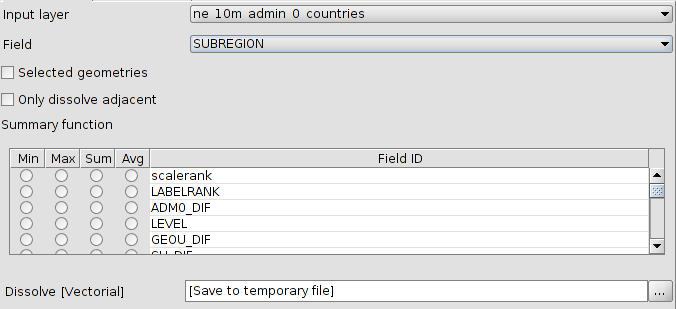1. Input layer. To select the layer to dissolve.

2. Field. To select the attribute to be used as the criteria for for fusionating the features of the layer.

3. Selected features. Option to use only the selected features in both layers.

5. Summary function. It is possible to apply a summary function over the numeric values of the linked features: Minimum(Min), maximum (Max), summation (Sum) and average (Avg). A field will be created for each function in the output layer.

When the geoprocess finishes, the new layer will be included in the TOC.

## 41.10. Fuse spatially

This geoprocess works with one only input layer, whose geometry type must be polygon. This process analyse each input geometry and builds a unique polygon with all geometries which intersects each other. The attribute table will have an identifier field of each feature. Furthermore, other layer with only alphanumeric data will be created. This alphanumeric table will contain the table of the input layer and it will include an ID field with a reference to the polygon in the first layer. In this way, each polygon in the first layer will have a reference to each feature in the second layer.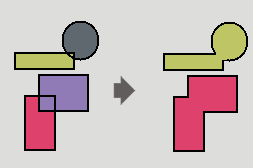The interface is: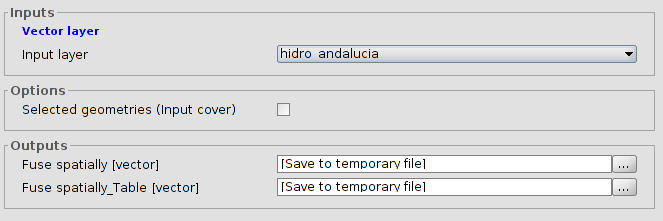1. Input layer.

2. Selected features (input layer). Option to use only the selected features in both layers.

## 41.11. Intersection

This geoprocess works with two layers: the input layer and the overlay layer. For each geometry in the input layer, it computes its intersections with the geometries of the overlay layer. For each intersection, it adds a new feature with the intersection as geometry and all attributes of the features which originates it. It is called "Spatial AND", because features of the result layer models a space common to both layers.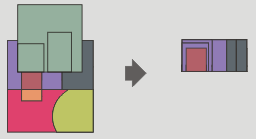The interface is: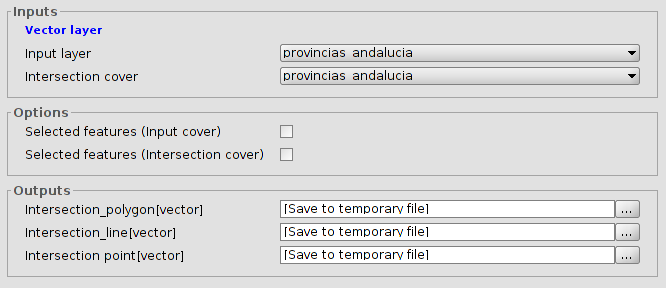1. Input layer.

2. Intersection cover. To select the intersection layer.

3. Selected features. Option to use only the selected features in both layers.

The type of the output layer will depend on the input layer:

• Between a polygon layer and a linear one, the output layer will be polygonal.

• Between a polygon layer and a point one, the output layer will be polygonal.

• Between two linear layers, the output layer will be a point layer, with the crosspoints between the lines of both layers.

• Between two polygonar layers, the output layer will be another polygonal layer.

## 41.12. Merge

This geoprocess can work with many input layers. It merges all of these creating a new result layer which has a feature for each feature of all input layers.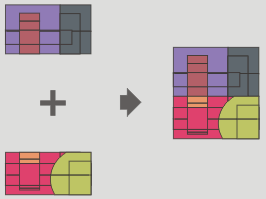The interface is: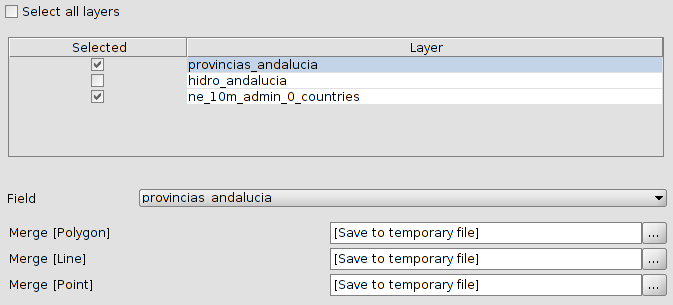1. Selection of layers for the merge.

2. Field. Because each input layer could have its own alphanumeric schema, user must select which layer will preserve its schema. The rest of input layers will only preserve those attributes whose name and type would be the same that an attribute of the input layer whose schema will be preserved.

When the geoprocess finishes, the new layer will be included in the TOC.

## 41.13. Reproject

This geoprocess will change the CRS of the input layer.

The interface is: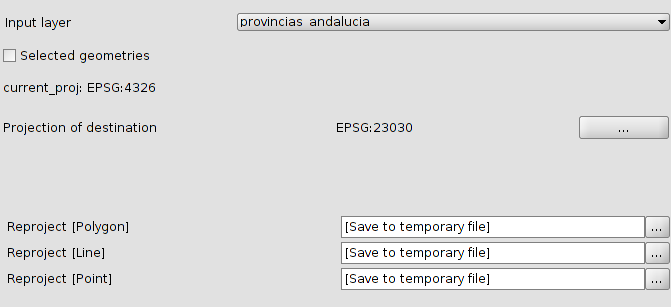1. Input layer to reproject.

2. Selected features. Option to use only the selected features.

3. Projection of destination. Use “...” to set the projection for the new layer.

## 41.14. Spatial Join

This geoprocess transfer attributes from a source layer to a target layer (very similar to an alphanumerical join). The difference with a traditional join is that the transfer criteria is not the value of one (or many) given attributes.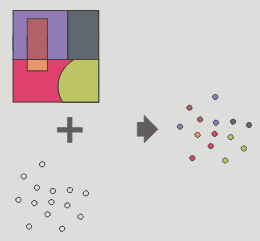Permite seguir dos tipos de criterios espaciales para establecer el enlace espacial:

Vecino más próximo (relación 1->1). Asigna a un elemento de la capa origen, los atributos del elemento más próximo de la capa enlazada. En caso de que el elemento más próximo intersecte (o esté contenido para el caso de polígonos) al elemento original, el algoritmo tomará el primer elemento analizado de las posibles intersecciones.

Contenido en (relación 1->M). Relaciona un elemento de la capa origen con varios elementos de la capa destino (en concreto, con aquellos que son intersectados). En este caso la capa origen no heredará los atributos de la capa relacionada, sino que la operativa será muy parecida a la del geoproceso “Disolver”. Para los M elementos relacionados con un elemento de la capa origen, se dará al usuario la posibilidad de escoger una o varias funciones resumen (media, mínimo, máximo, sumatorio) que se aplicarán sobre los atributos numéricos de la capa enlazada.

The interface is: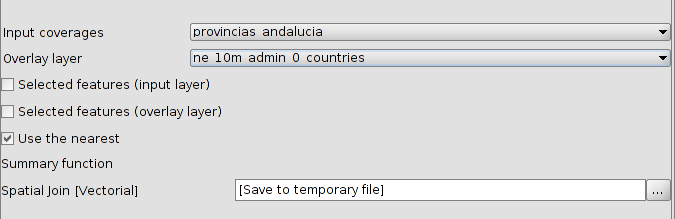1. Input layer.

2. Overlay layer.

3. Selected features. Option to use only the selected features in both layers.Geometrías seleccionadas.

4. Use nearest. Permite seleccionar una relación 1-1 mediante el criterio espacial de vecino más próximo. Si no se activa la casilla de verificación se aplica una relación 1-N, mediante el criterio espacial 'Intersecta' o 'Contenido en'.

5. Summary function. Permite aplicar una función resumen sobre los atributos numéricos de los elementos enlazados. Las funciones resumen soportadas son mínimo (Min), máximo (Max), sumatorio (Sum) y media (Avg). Se incluirá un campo en la capa resultado para cada función resumen seleccionada.

When the geoprocess finishes, the new layer will be included in the TOC.

## 41.15. Union

Like Intersect and Difference, this geoprocess is an overlay geoprocess. It works with an input and an overlay layer; it is also called "Spatial OR" because features of the result layer will have features whose geometry is in any of the input or the overlay layer. This means that the geoprocess takes three steps:

• The first one to calculate the intersection of both tables.

• The second one to calculate the differences between the first and the second one.

• The third one to calculate the differences between the second and the first one.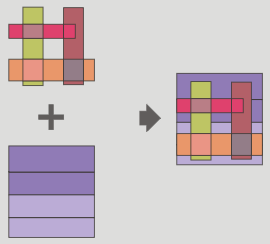The interface is: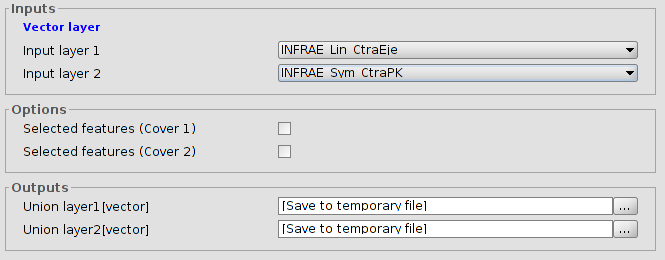1. Input layer. To select the layers.

2. Selected features. Option to use only the selected features.

The results of unions with different geometries are:

• Between a polygonal layer and one point layer, the output layers will be one point layer and another polygonal layer.

• Between a polygonal layer and one linear layer, the output layers will be one linear layer and another polygonal layer.

• Between two linear layers, the output layers will be one linear layer and another point layer with the intersection between them.